# Solving Equations Using Multiplication or Division

##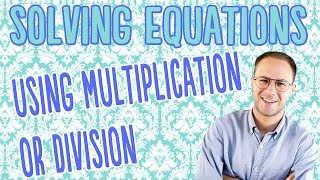By Anywhere Math# Division - Sharing Equally: Grade 3

##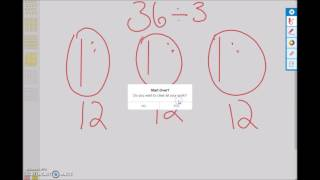By university of Houston mathematics education

Use multiplication and division within 100 to solve word problems in situations involving equal groups, arrays, and measurement quantities, e.g., by using drawings and equations with a symbol for the unknown number to represent the problem.# Division - Sharing Equally: Grade 3

##By university of Houston mathematics education

Use multiplication and division within 100 to solve word problems in situations involving equal groups, arrays, and measurement quantities, e.g., by using drawings and equations with a symbol for the unknown number to represent the problem.# Division Problem Solving

##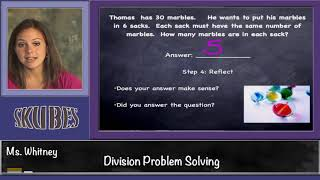By Skubes ed

Use multiplication and division within 100 to solve word problems in situations involving equal groups, arrays, and measurement quantities, e.g., by using drawings and equations with a symbol for the unknown number to represent the problem.1# One-step equations with multiplication and division

##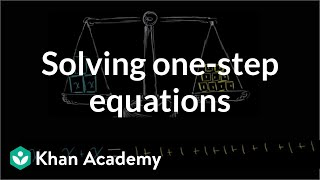By Khan Academy

This equation can be simplified through a single step to solve for the variable. Can you help?# One-step equations with multiplication and division

##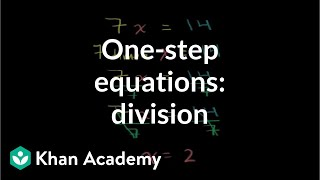By Khan Academy

Let's ease into this, shall we? Here's an introduction to basic algebraic equations of the form ax=b. Remember that you can check to see if you have the right answer by substituting it for the variable!# One-step equations with multiplication and division

##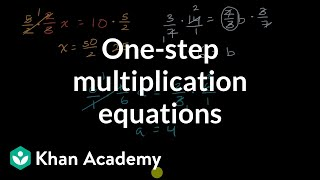By Khan Academy

Remember that what you do to one side, you have to do to the other. Will you multiply or divide both sides to dump the fraction, x/a? Let's do it together.# One-step equations with multiplication and division

##By Khan Academy

Let's get a conceptual understanding of why one needs to divide both sides of an equation to solve for a variable.# One-step equations with multiplication and division

##By Khan Academy

Let's ease into this, shall we? Here's an introduction to basic algebraic equations of the form ax=b. Remember that you can check to see if you have the right answer by substituting it for the variable!# One-step equations with multiplication and division

##By Khan Academy

Remember that what you do to one side, you have to do to the other. Will you multiply or divide both sides to dump the fraction, x/a? Let's do it together.# One-step equations with multiplication and division

##By Khan Academy

This equation can be simplified through a single step to solve for the variable. Can you help?# One-step equations with multiplication and division

##By Khan Academy

Let's get a conceptual understanding of why one needs to divide both sides of an equation to solve for a variable.# Composing equations and inequalities

##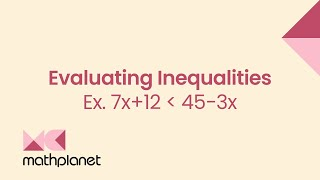By MathPlanetVideos

Is 3 a solution to 7x+12<45−3x# Understanding inequalities and equations

##By MathPlanetVideos

Margaret is paying \$1,500 in rent for her apartment every month. Margaret won \$12,000 in Vegas and wondering how many rent she can afford to pay with this winning?# Reasoning with Equations and Inequalities

##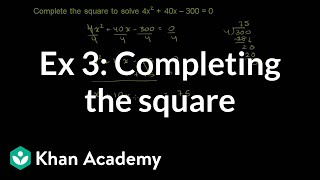By Khan Academy

Sal solves the equation 4x^2+40x-300=0 by completing the square.265# Modeling with one-variable equations and inequalities

##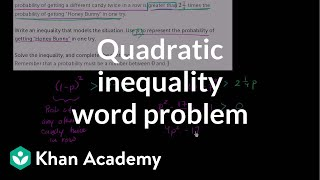By Khan Academy

Sal models a context that concerns a candy vending machine. The model turns out to be a quadratic inequality.# Modeling with one-variable equations and inequalities

##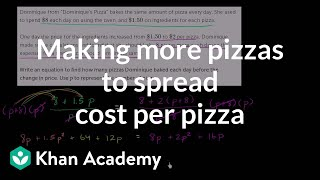By Khan Academy

Sal models a context that concerns the number and price of pizza slices. The model turns out to be a rational equation.# Modeling with one-variable equations and inequalities

##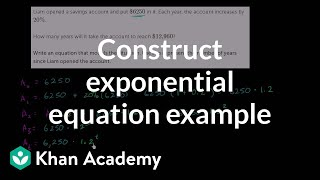By Khan Academy

Sal models a context that concerns a bank savings account. The model turns out to be an exponential equation.# One-step multiplication and division equations with fractions and decimals

##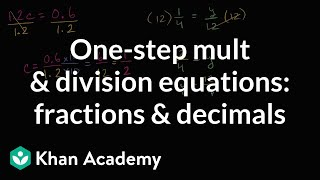By Khan Academy

Learn how to solve equations in one step by multiplying or dividing a number from both sides.ï¿½ï¿½ï¿½ï¿½ï¿½ï¿½ï¿½ï¿½ï¿½ï¿½ï¿½ï¿½These problems involve decimals and fractions.# One-Step Division Equations - MathHelp.com - Math Help

##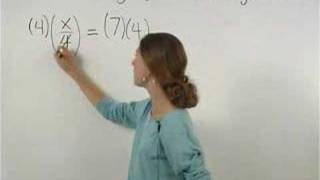By yourteachermathhelp

1000+ online math lessons featuring a personal math teacher inside every lesson! In this lesson students learn to solve one-step division equations. For example to solve x/4 = 2 multiply both sides of the equation by 4 to get x = 8. Next check the solution by substituting an 8 back into the original equation to get (8)/2 = 4 which is a true statement so the solution checks.